# 7 An object of mass 2.00 kg starts at rest from the top of a tough...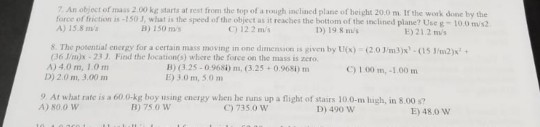7 An object of mass 2.00 kg starts at rest from the top of a tough inclned plane of height 20.0 m. If the work done by the force of friction as-150 3, what is the speed of the object as it reaches the botiom of the inclined plane? Use g- 1o.0 m/s A) 15.8 m B) 150 ros C) 12 2 ms D) 19 8 ms E) 21.2 ms S. The posential energy for a certain mass moving in one dimensaon s given by tlex) -(203m3)x-(15 m2) G6 m)x -23 Find the location(s) where the force on the mass is zero A) 4.0 m, 1.0m B) (3.25-0968i) m, (3.25+0.9681) m E) 3.0m, 50m C) 1 00 m, -1.00m D) 2.0 m, 3.00m 9 At what rate is a 60 0-kg boy ising energy when he nins up a flight of stars 100-m ¡ugh, in 8.00 s? A) 800 W B) 75 0 W ) 735.0 Vw D) 490 W E)480 W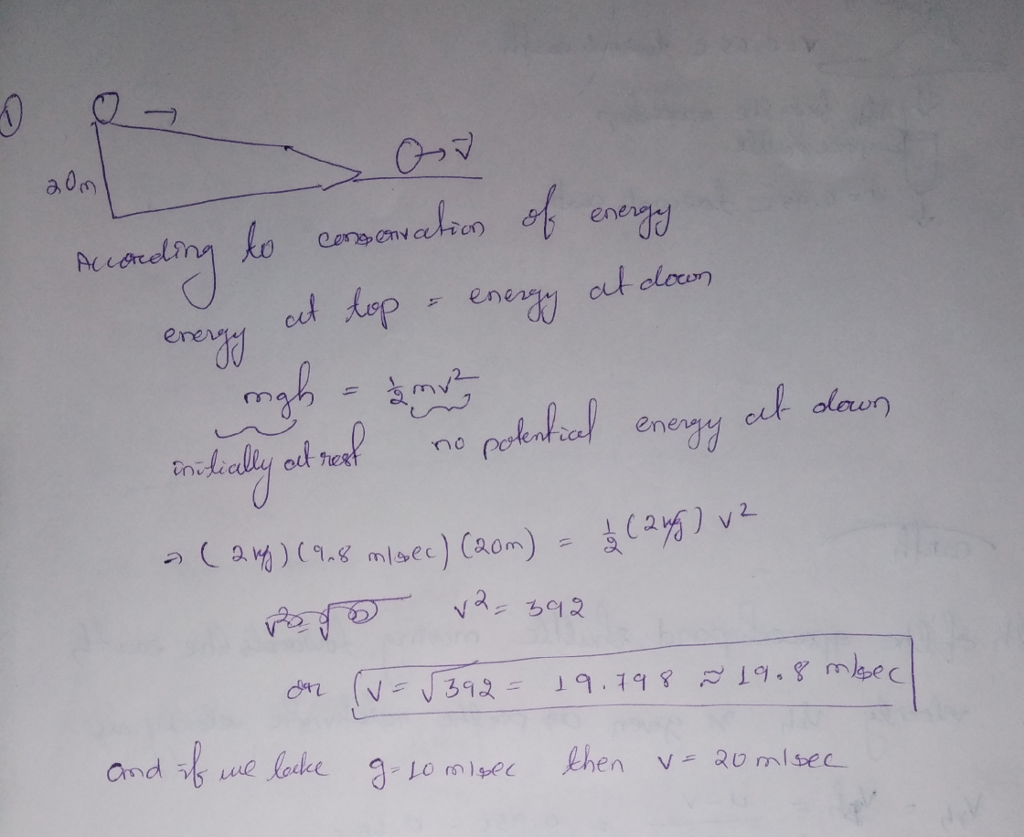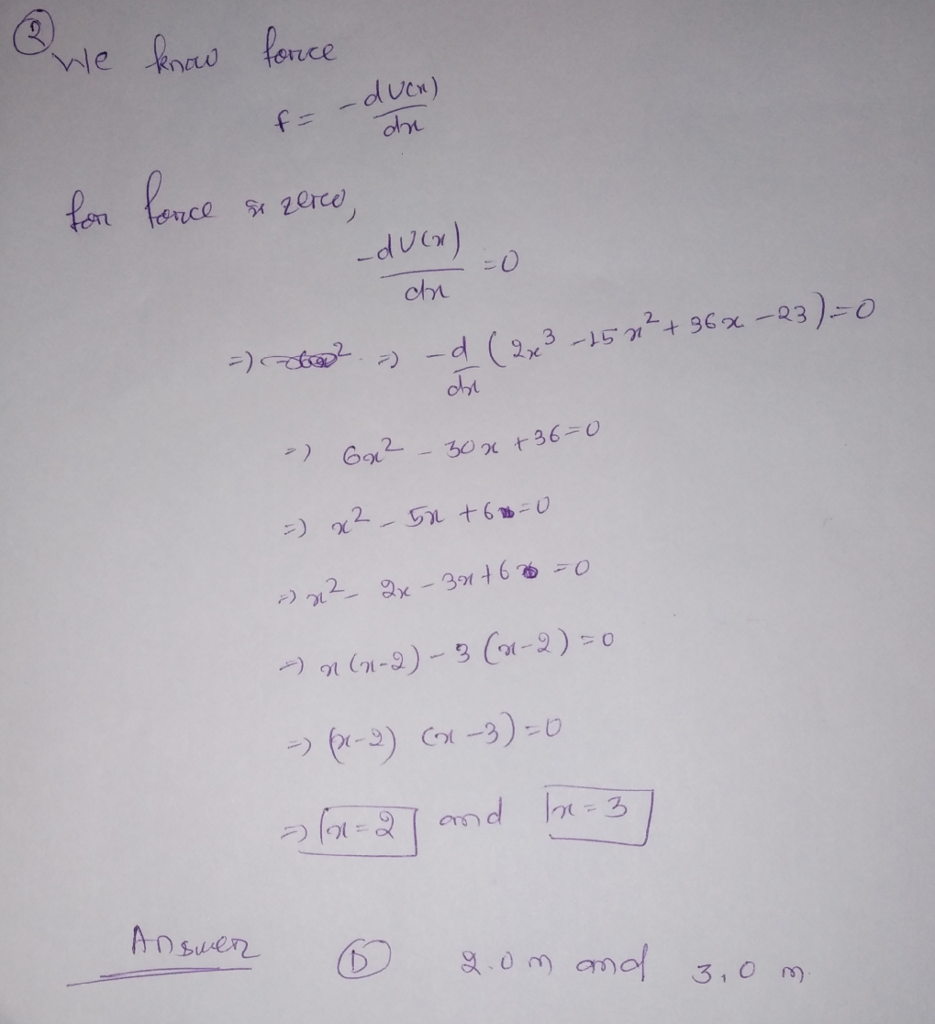#### Earn Coin

Coins can be redeemed for fabulous gifts.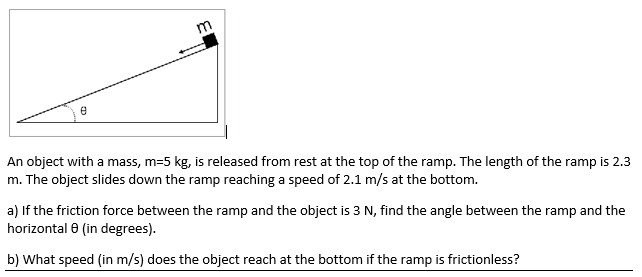An object with a mass, m = 5 kg, is released from rest at the top of the ramp. The length of the ramp is 2.3 m. The object slides down the ramp reaching a speed of 2.1 m/s at the bottom. a) If the friction force between the ramp and the object is 3 N, find the angle between the ramp and the horizontal theta (in degrees). b) What speed (in m/s) does the object reach at the bottom...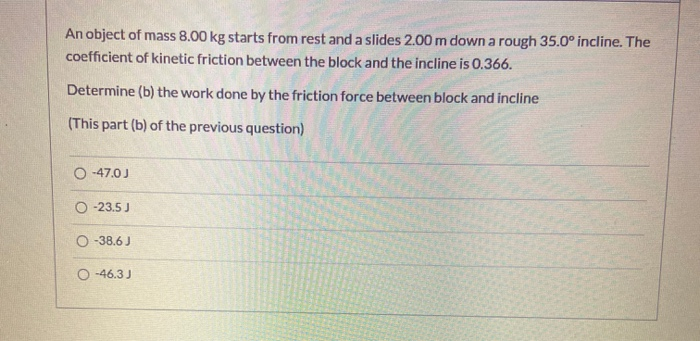An object of mass 8.00 kg starts from rest and a slides 2.00 m down a rough 35.0° incline. The coefficient of kinetic friction between the block and the incline is 0.366. Determine (b) the work done by the friction force between block and incline (This part (b) of the previous question) 0 -47.00 0 -23.5) 0 -38.6) O-46.3)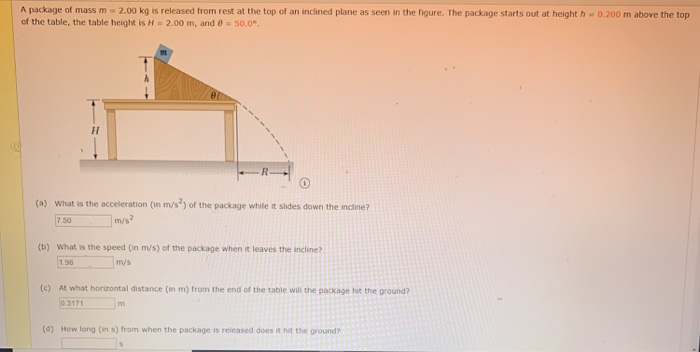A package of mass m = 2.00 kg is released from rest at the top of an inclined plane as seen in the figure. The package starts out at heighth = 0.200 m above the top of the table, the table height is H = 2.00 m, and 0 = 50.0° R- (a) What is the acceleration (in m/s) of the package while it slides down the incline? 7.50 m/s² (b) What is the speed (in m/s) of the package...